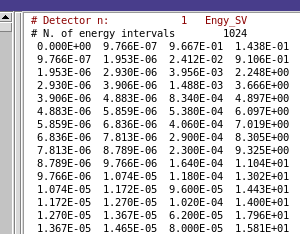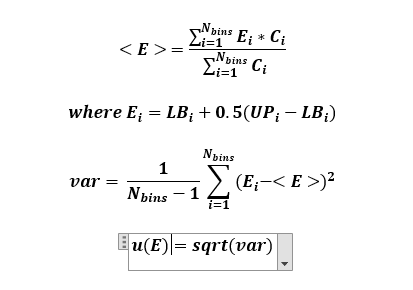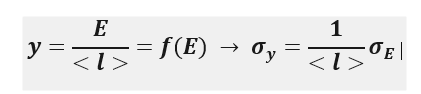# Uncertainty: Overall Value

Dear FLUKA experts,
I’m running a FLUKA simulation to calculate energy deposition in Tissue Equivalent gases using the DETECT Card,

The “-tab.lis” file outputs 4 columns, which I understand to be [LB,UB, C, u(%)] where,
LB = lower energy bin,
UB = upper energy bin,
C = Counts or pulse height spectrum and
u = uncertainty per energy bin
as shownQuestion 1:
How do I calculate the overall uncertainty of the average energy imparted in the gas? Do the u-values play a role in this?

I understand that “u” is calculated as shown here (chrome-extension://efaidnbmnnnibpcajpcglclefindmkaj/https://indico.cern.ch/event/1123370/contributions/4715934/attachments/2444331/4188477/02_Monte_Carlo_Basics_2022_ULB.pdf)

I calculated the average energy imparted and the uncertainty byIs that correct? This doesn’t include the u-values though.

Question 2:
Is there a possibility to define logarithmic energy bins within the detect card?
Zavier Ndum

Dear Zavier,

1. I don’t think you can use the same formula, since the bins are different “quantities”, not multiple measurements of the same one.

To calculate the error of the average energy, you can use the standard propagation of uncertainty (Propagation of uncertainty - Wikipedia). But it would be much easier, just to add a standard FLUKA scoring for energy deposition (USRBIN - Regions)

2. It is not possible to have logarithmic binning for the DETECT scoring.

Cheers,
David

Dear David,
I understand that I cannot use the formula posted in the second picture since that corresponds to the uncertainty of the counts (C_i). But what about the third picture of how I calculated the average energy deposited in the target, with the variance and uncertainty?
Doesn’t this agree with the statement from the link you sent on uncertainty propagation? “… Most commonly, the uncertainty on a quantity is quantified in terms of the standard deviation, σ, which is the positive square root of the variance.”

My understanding of uncertainty propagation is that if I were to calculate the uncertainty of another quantity related to the energy like the lineal energy (y), then I could proceed as followsCorrect? But how do I get sigma_E of the raw distribution and understanding If have to utilize the uncertainty of the counts from FLUKA and how to do that is my problem?

Also, I do not understand what you mean by “… But it would be much easier, just to add a standard FLUKA scoring for energy deposition (USRBIN - Regions)”. Does this mean I’ll have to define a USRBIN in the target and separately score the average energy deposited in this region? Different from the DETECT card?

Zavier N. Ndum

Dear Zavier,

using the formula on your third picture doesn’t make sense, since the E_i values are not from a “random variable”.

To calculate the error of the average energy, you need to propagate the separate \sigma_{C_i} errors.

You can define a separate USRBIN scoring, giving you the average energy deposition (with the correct error) per primary particle.
If you want the average energy per energy deposition in the detector you could use the EVENTBIN scoring. This will give you the total deposited energy in the detector for each primary particle, but it would require some manual post-processing to get the average value and error.

Cheers,
David